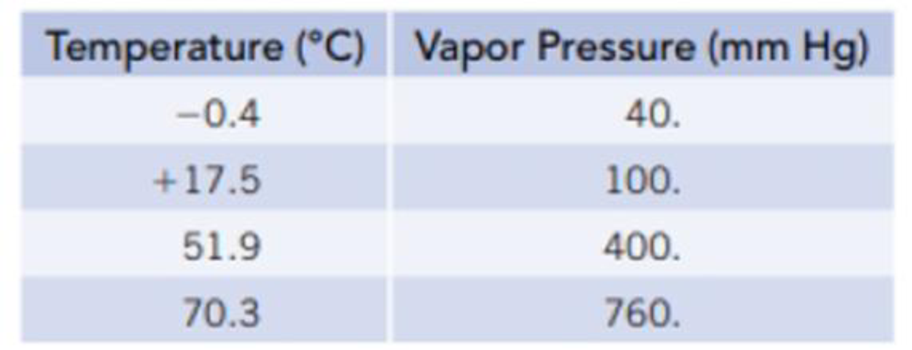# You are going to prepare a silicone polymer, and one of the starting materials is dichlorodimethylsilane, SiCl 2 (CH 3 ) 2 . You need its normal boiling point and to measure equilibrium vapor pressures at various temperatures. (a) What is the normal boiling point of dichlorodimethylsilane? (b) Plot these data as ln P versus 1/ T so that you have a plot resembling the one in Figure 11.13. At what temperature does the liquid have an equilibrium vapor pressure of 250 mm Hg? At what temperature is it 650 mm Hg? (c) Calculate the molar enthalpy of vaporization for dichlorodimethylsilane using the Clausius-Clapeyron equation.### Chemistry & Chemical Reactivity

10th Edition
John C. Kotz + 3 others
Publisher: Cengage Learning
ISBN: 9781337399074### Chemistry & Chemical Reactivity

10th Edition
John C. Kotz + 3 others
Publisher: Cengage Learning
ISBN: 9781337399074

#### Solutions

Chapter
Section
Chapter 11, Problem 39IL
Textbook Problem

## You are going to prepare a silicone polymer, and one of the starting materials is dichlorodimethylsilane, SiCl2(CH3)2. You need its normal boiling point and to measure equilibrium vapor pressures at various temperatures.(a) What is the normal boiling point of dichlorodimethylsilane? (b) Plot these data as ln P versus 1/T so that you have a plot resembling the one in Figure 11.13. At what temperature does the liquid have an equilibrium vapor pressure of 250 mm Hg? At what temperature is it 650 mm Hg? (c) Calculate the molar enthalpy of vaporization for dichlorodimethylsilane using the Clausius-Clapeyron equation.

Expert Solution

(a)

Interpretation Introduction

Interpretation:

The normal boiling point of dichlorodimethylsilane has to be determined

Concept Introduction:

Boiling point of a liquid: The temperature at which external pressure and vapour pressure of the liquid become same.

Normal boiling point: When the external pressure is 760mmHg we can call it as normal boiling point.

### Explanation of Solution

The normal boiling point of dichlorodimethylsilane is calculated

Given:

Temperature(K)VapourPressure(mmHg)272

Expert Solution

(b)

Interpretation Introduction

Interpretation:

The graph of lnP versus 1/T has to be plotted. The temperatures at which liquid have a vapour pressures of 250mmHg and 650mmHg has to be determined.

Concept Introduction:

Clausius-Clapeyron equation:

lnP=(ΔvapH0RT)+C

From this relationship we can calculate the molar enthalpy of vaporization by knowing the corresponding temperature and pressure values.

If we have pressures at two different temperatures, then enthalpy of vaporization can be calculated by

lnP2p1=-ΔvapH0R[1T2-1T1]

Expert Solution

(c)

Interpretation Introduction

Interpretation:

The molar enthalpy of vaporization has to be explained.

Concept Introduction:

Clausius-Clapeyron equation:

lnP=(ΔvapH0RT)+C

From this relationship we can calculate the molar enthalpy of vaporization by knowing the corresponding temperature and pressure values.

If we have pressures at two different temperatures, then enthalpy of vaporization can be calculated by

lnP2p1=-ΔvapH0R[1T2-1T1]

Boiling point of a liquid: The temperature at which external pressure and vapour pressure of the liquid become same.

Normal boiling point: When the external pressure is 760mmHg we can call it as normal boiling point.

Molar enthalpy of vaporization: The energy required to convert liquid to gas of 1mol of a substance is called molar enthalpy of vaporization

### Want to see the full answer?

Check out a sample textbook solution.See solution

### Want to see this answer and more?

Bartleby provides explanations to thousands of textbook problems written by our experts, many with advanced degrees!

See solution